跳表的设计和实现

流程

[0,20000]

[5, 43, 6, 1, 3, 8, 5]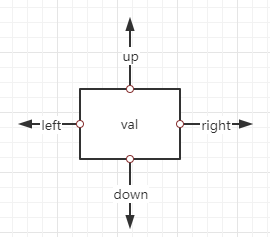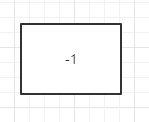a -> 5 -> c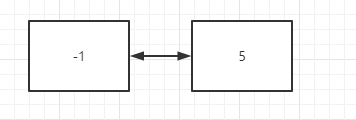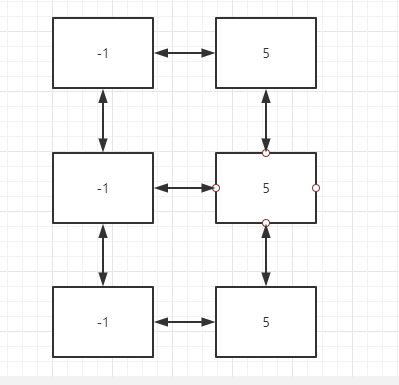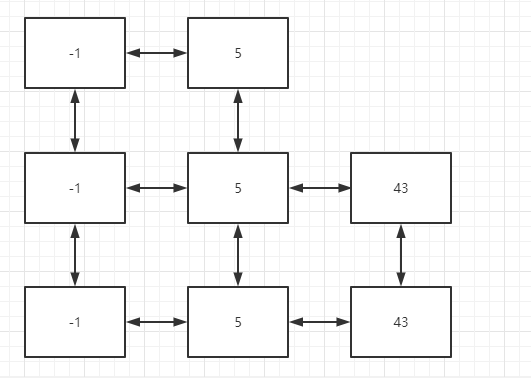4已经超过了目前的最大层数3，所以，要扩充头节点链表，且6必须插在5和43之间，所以，6这个元素加入到跳表后，跳表的样子如下：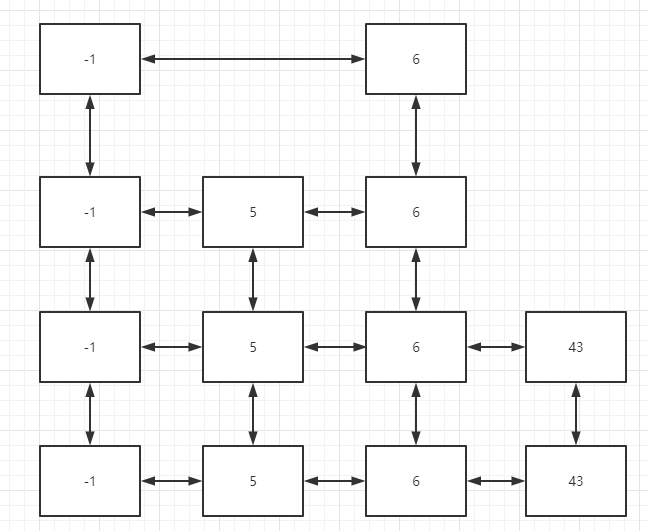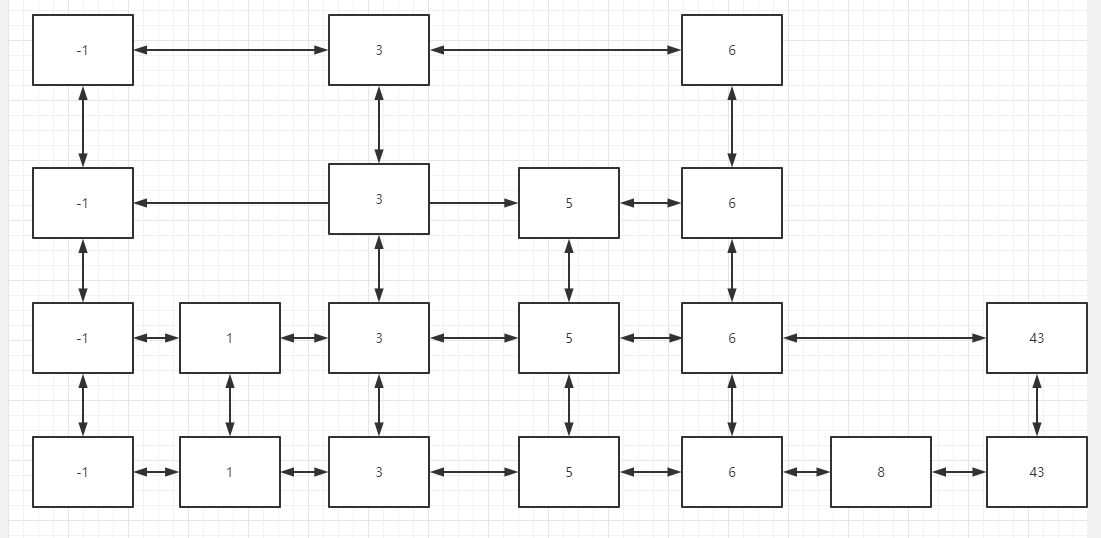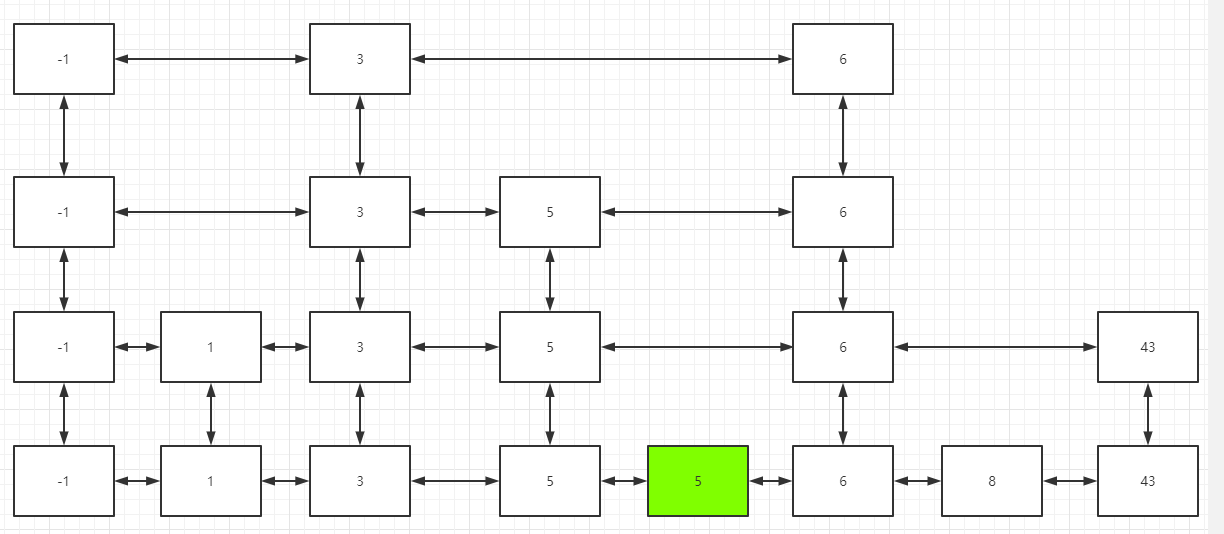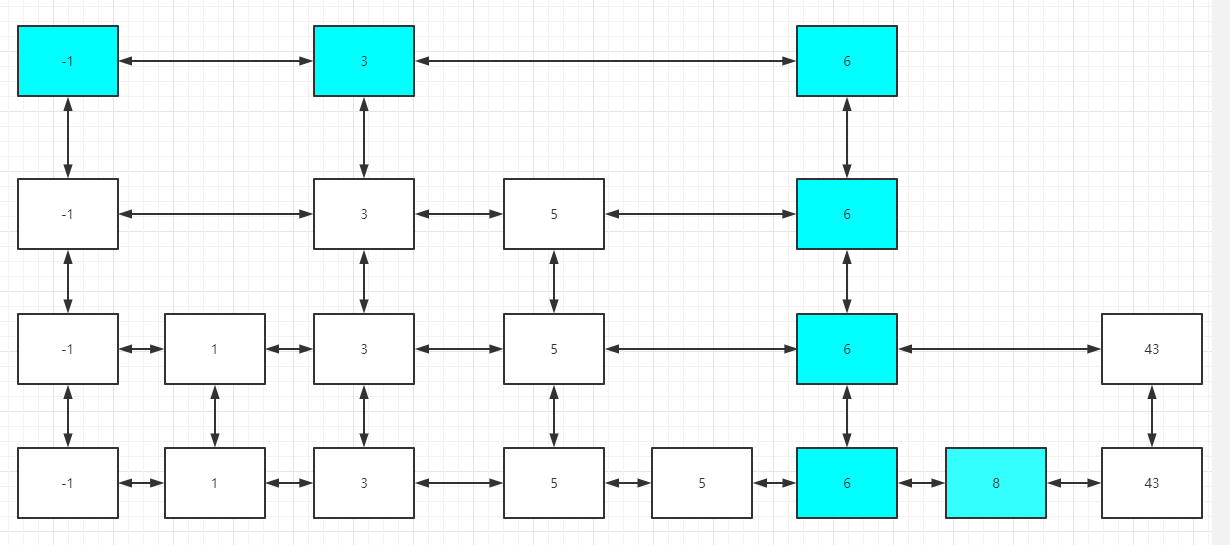1. 找到a这个节点最底层的位置，如果找不到，直接不需要操作删除。

2. 找到a这个节点在的底层位置，依次往上（node = node.up），删掉每一个元素，直到不能往上为止。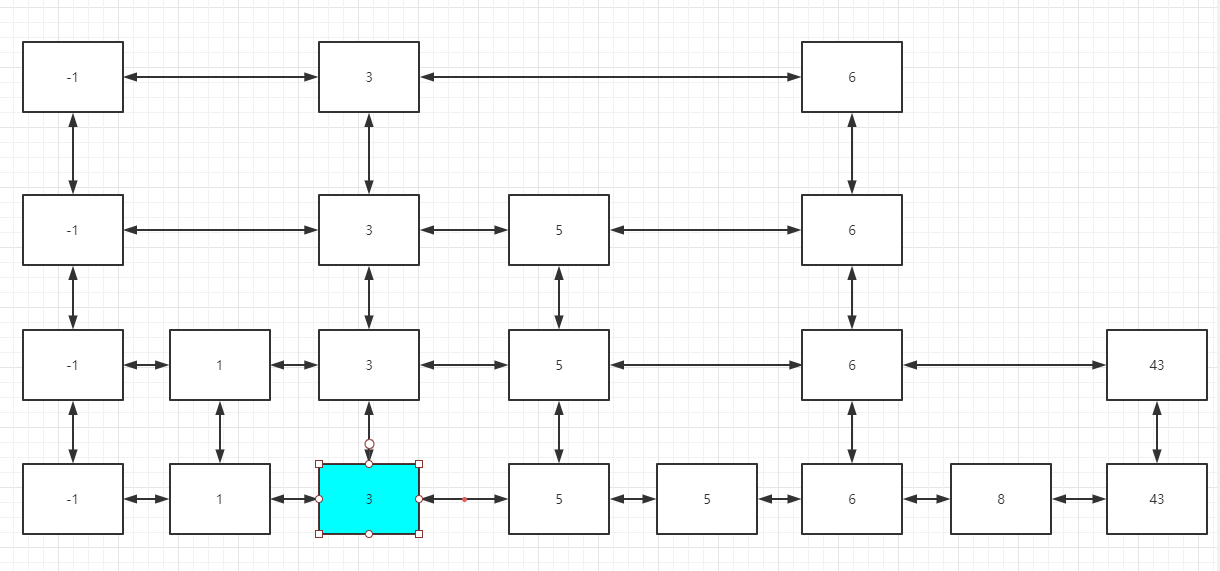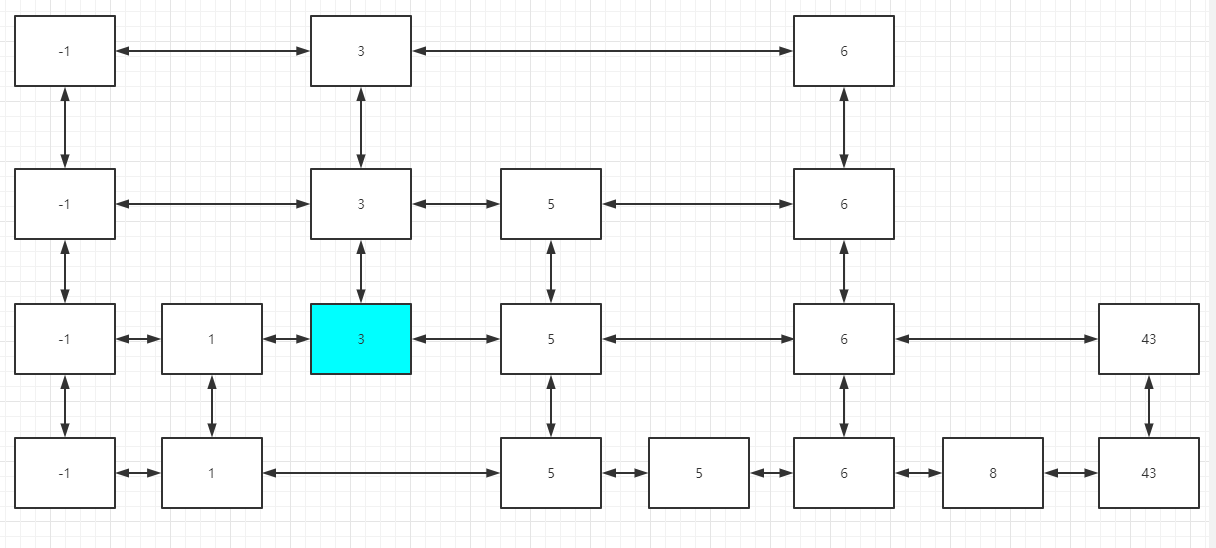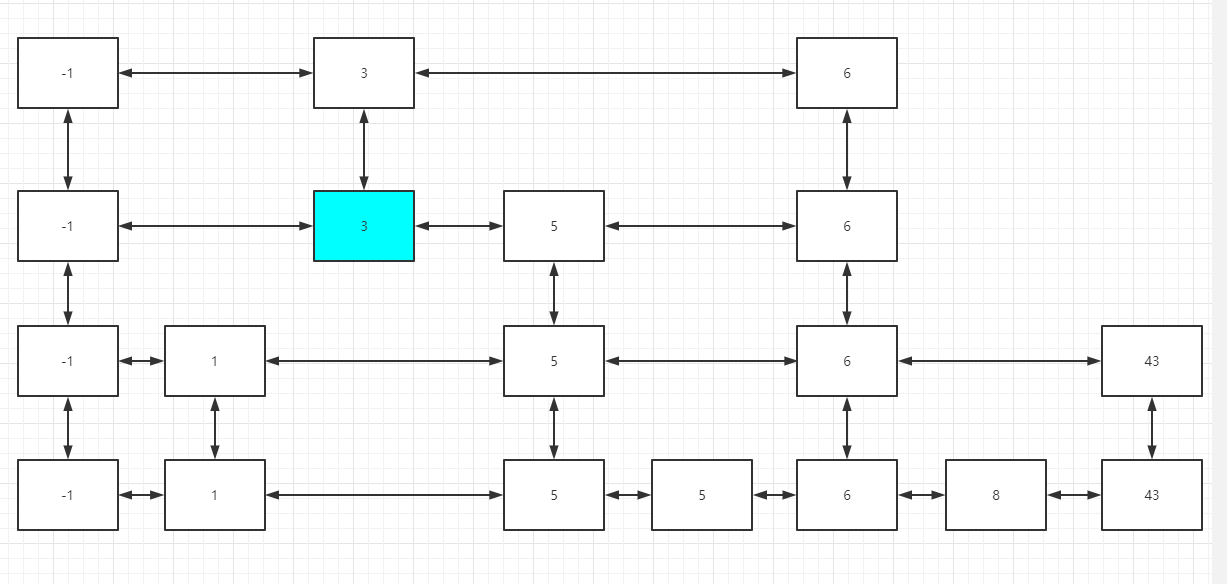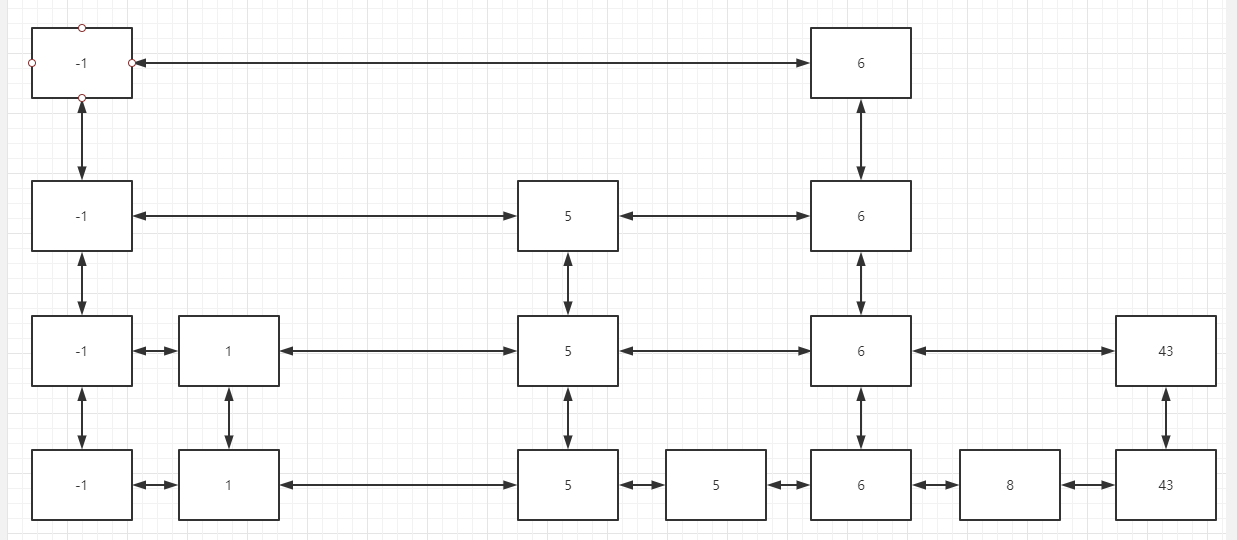细节

随机生成层数

// < 0.5 : 增加一层，
// >= 0.5 ：不再增加
public boolean roll() {
return Math.random() < 0.5d;
}

获取跳表中小于或某个元素的最右位置的元素

public Node getLessOrEqual(int target) {
// 获取头节点的最顶端的位置的那个元素
while (cur != null) {
// 如果比目标值小，就一直向右边移动（在右边不为空的情况下），如果右边为空了，继续向下移动，直到移动到最底端。
if (cur.right == null || cur.right.val > target) {
if (cur.val <= target) {
if (cur.down != null) {
cur = cur.down;
} else {
break;
}
}
} else {
cur = cur.right;
}
}
return cur;

}

完整代码

class Skiplist {
private final static double POSSIBLE = 0.5d;

public Skiplist() {
}

public static class Node {
private int val;
private Node up, down, left, right;

public Node(int val) {
this.val = val;
}
}

public Node getLessOrEqual(int target) {
while (cur != null) {
if (cur.right == null || cur.right.val > target) {
if (cur.val <= target) {
if (cur.down != null) {
cur = cur.down;
} else {
break;
}
}
} else {
cur = cur.right;
}
}
return cur;

}

public boolean search(int target) {
return getLessOrEqual(target).val == target;
}

public boolean roll() {
return Math.random() < POSSIBLE;
}

// 如果节点存在则不增加
Node lessOrEqual = getLessOrEqual(num);
// 支持重复数据插入，所以这里不能直接判断存在就退出
/*  if (lessOrEqual.val == num) {
return;
}*/

// 到这里说明节点不存在，先建出节点
Node newNode = new Node(num);

// 无论如何，最底层都要连起来的
Node right = lessOrEqual.right;
lessOrEqual.right = newNode;
newNode.left = lessOrEqual;
if (right != null) {
// lessOrEqual不是最后一个节点
newNode.right = right;
right.left = newNode;
}

// 掷骰子随机确定其他的层数
Node pre = lessOrEqual;
Node cur = newNode;
while (roll()) {
while (pre.left != null && pre.up == null) {
pre = pre.left;
}
if (pre.left == null) {
final Node head = new Node(-1);
}
pre = pre.up;
Node toInsert = new Node(num);
cur.up = toInsert;
toInsert.down = cur;
cur = cur.up;
pre.right = toInsert;
cur.left = pre;
}
}

public boolean erase(int num) {
Node lessOrEqual = getLessOrEqual(num);
if (lessOrEqual.val != num) {
return false;
}

Node cur = lessOrEqual;
while (cur != null) {
Node pre = cur.left;
Node after = cur.right;

pre.right = after;
if (after != null) {
after.left = pre;
}
cur = cur.up;
}
return true;
}
}

跳表的应用

Redis底层使用了跳表，为什么选跳表而不是AVL树或者SBT红黑树来实现，因为跳表方便序列化。

参考资料

posted @ 2021-05-13 12:48  Grey Zeng  阅读(263)  评论(0编辑  收藏  举报